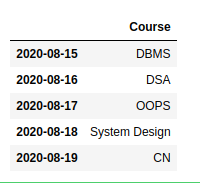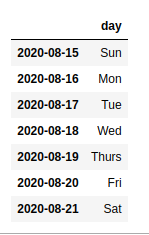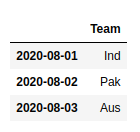# Determine Period Index and Column for DataFrame in Pandas

• Difficulty Level : Medium
• Last Updated : 11 Jun, 2021

In Pandas to determine Period Index and Column for Data Frame, we will use the pandas.period_range() method. It is one of the general functions in Pandas that is used to return a fixed frequency PeriodIndex, with day (calendar) as the default frequency.

Syntax: pandas.to_numeric(arg, errors=’raise’, downcast=None)

Parameters:
start : Left bound for generating periods
end : Right bound for generating periods
periods : Number of periods to generate
freq : Frequency alias
name : Name of the resulting PeriodIndex

Returns: PeriodIndex

Example 1:

## Python3

 `import` `pandas as pd`   `course ``=` `[``"DBMS"``, ``"DSA"``, ``"OOPS"``,``          ``"System Design"``, ``"CN"``, ]` `# pass the period and starting index``webinar_date ``=` `pd.period_range(``'2020-08-15'``, periods``=``5``)` `# Determine Period Index and Column``# for DataFrame``df ``=` `pd.DataFrame(course, index``=``webinar_date, columns``=``[``'Course'``])` `df`

Output:Example 2:

## Python3

 `import` `pandas as pd` `day ``=` `[``"Sun"``, ``"Mon"``, ``"Tue"``,``       ``"Wed"``, ``"Thurs"``, ``"Fri"``, ``"Sat"``]` `# pass the period and starting index``daycode ``=` `pd.period_range(``'2020-08-15'``, periods``=``7``)` `# Determine Period Index and Column for DataFrame``df ``=` `pd.DataFrame(day, index``=``daycode, columns``=``[``'day'``])` `df`

Output:Example 3:

## Python3

 `import` `pandas as pd` `Team ``=` `[``"Ind"``, ``"Pak"``, ``"Aus"``]` `# pass the period and starting index``match_date ``=` `pd.period_range(``'2020-08-01'``, periods``=``3``)` `# Determine Period Index and Column for DataFrame``df ``=` `pd.DataFrame(Team, index``=``match_date, columns``=``[``'Team'``])` `df`

Output:My Personal Notes arrow_drop_up